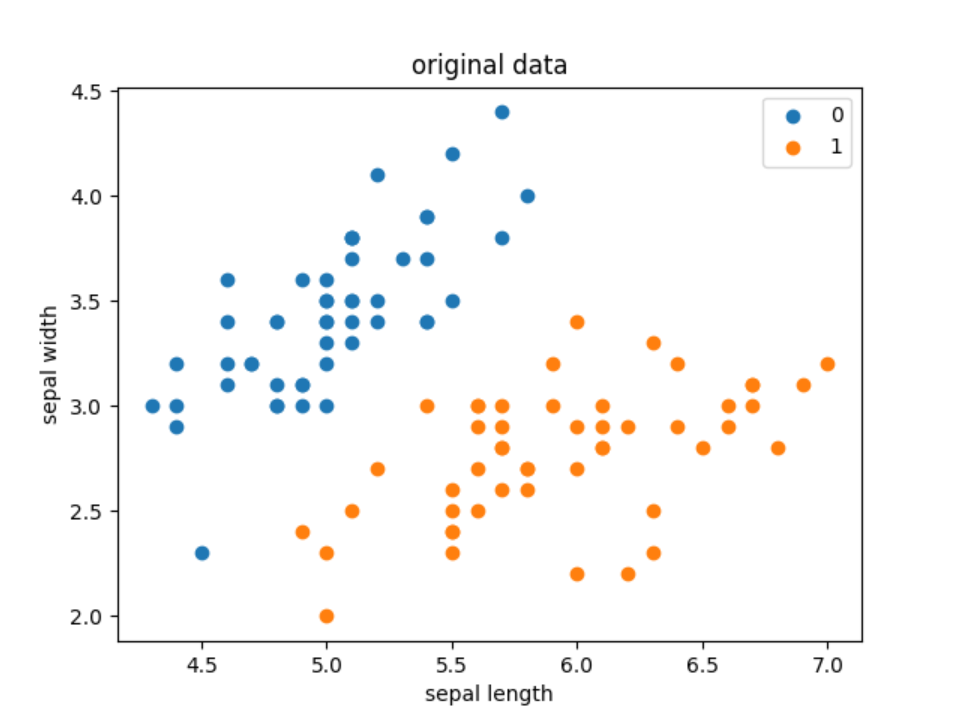# 机器学习-感知机模型 - 叶小小qaq

Go to the source link to view the article. You can view the picture content, updated content and better typesetting reading experience. If the link is broken, please click the button below to view the snapshot at that time.

单层感知机是神经网络的一个基本单元，类似于人类的神经网络的一个神经元，神经网络是由具有适应性的简单单元(感知机)组成的广泛并行互连的网络，它的组织能够模拟生物神经系统对真实世界物体所作出的交互反应。感知机可以理解为对输入进行处理，并得到输出结果的机器。我们似乎明白了很多，但是不明白到底为什么明白，就和人的大脑一样神秘。

感知机1957年由Rosenblatt提出，是神经网络与支持向量机的基础。是二类分类的线性分类模型，属于判别模型，旨在求出将训练数据进行线性划分的分离超平面，为此，导入基于误分类的损失函数，利用梯度下降法对损失函数进行极小化，求得感知机模型。

• 训练数据集：线性可分（必存在超平面将训练集正负实例分开）
• 学习目标：找到一个将训练集正、负实例点完全正确分开的超平面
• 具体学习对象：• 学习策略：误分类点到超平面S的总距离最小
• 算法形式：原始形式+对偶形式

## 二、从机器学习的角度认识感知机

### 1.感知机模型

• 输入空间（特征空间）是，输入表示实例的特征向量
• 输出空间是，输出表示实例的类别。
• 输入空间 —> 输出空间：其中，为感知机模型参数，也就是我们机器学习最终要学习的参数

sign是符号函数，常用的经典形式，由于只有一层，又被称为单层感知机。如下：从几何角度分析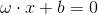相当于n维空间的一个超平面。ω为超平面的法向量，b为超平面的截距，x为空间中的点。

当x位于超平面的正侧时：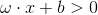感知机被激活。

当x位于超平面的负侧时：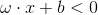感知机被抑制。

所以，从几何的角度来看，感知机就是n维空间的一个超平面，它把特征空间分成两部分。

### 2.分类与学习策略### 3.原始算法

下面我们来看感知机的学习算法。给定一个训练数据集T={(x1,y1),(x2,y2),...,(xN,yN)}T={(x1,y1),(x2,y2),...,(xN,yN)}

1.假设误分类点的集合为 M，那么损失函数L(w, b)的梯度为: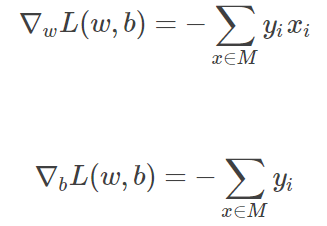2.随机选取一个误分类的点 (xi,yi)(xi,yi)，对 w, b 更新: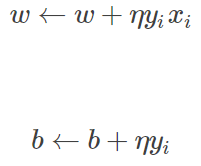### 4、算法——对偶形式• 输入：• 输出：1.2. 训练集中选取数据3. 如果4. 转至(2)，直至训练集中没有误分类点
5. 步骤解释：
6. 步骤1：和前面一样，初始值取0
7. 步骤2：每次也是选取一个数据
• 如果数据点是误判点的话，开始修正
• 这里关注一下梯度的计算：对于每一个数据每一次修正来说，的变化量是：，还是不变
• 步骤4：与原始形式一样，感知机学习算法的对偶形式迭代是收敛的，存在多个解

Gram matrix## 三、代码实现

`import pandas as pdimport numpy as npfrom sklearn.datasets import load_irisimport matplotlib.pyplot as plt #加载数据iris = load_iris()df = pd.DataFrame(iris.data, columns=iris.feature_names)df['label'] = iris.target df.columns = [ 'sepal length', 'sepal width', 'petal length', 'petal width', 'label']print(df.label.value_counts()) #画出原始数据离散图plt.scatter(df[:50]['sepal length'], df[:50]['sepal width'], label='0')plt.scatter(df[50:100]['sepal length'], df[50:100]['sepal width'], label='1')plt.xlabel('sepal length')plt.ylabel('sepal width')plt.title('original data')plt.legend()plt.show() data = np.array(df.iloc[:100, [0, 1, -1]])X, y = data[:,:-1], data[:,-1]print("X: ",X)print("y: ",y)y = np.array([1 if i == 1 else -1 for i in y]) #感知机模型# 数据线性可分，二分类数据# 此处为一元一次线性方程class Model: def __init__(self): self.w = np.ones(len(data) - 1, dtype=np.float32) self.b = 0 self.l_rate = 0.1 # self.data = data def sign(self, x, w, b): y = np.dot(x, w) + b return y # 随机梯度下降法 def fit(self, X_train, y_train): is_wrong = False while not is_wrong: wrong_count = 0 for d in range(len(X_train)): X = X_train[d] y = y_train[d] if y * self.sign(X, self.w, self.b) <= 0: self.w = self.w + self.l_rate * np.dot(y, X) self.b = self.b + self.l_rate * y wrong_count += 1 if wrong_count == 0: is_wrong = True return 'Perceptron Model!' def score(self): pass perceptron = Model()perceptron.fit(X, y) #画出训练结果x_points = np.linspace(4, 7, 10)y_ = -(perceptron.w * x_points + perceptron.b) / perceptron.wplt.plot(x_points, y_)plt.plot(data[:50, 0], data[:50, 1], 'bo', color='blue', label='0')plt.plot(data[50:100, 0], data[50:100, 1], 'bo', color='orange', label='1')plt.xlabel('sepal length')plt.ylabel('sepal width')plt.title('result')plt.legend()plt.show()`